﻿ The Discrete Poisson-Akash DistributionInternational Journal of Probability and Statistics

p-ISSN: 2168-4871    e-ISSN: 2168-4863

2017;  6(1): 1-10

doi:10.5923/j.ijps.20170601.01The Discrete Poisson-Akash Distribution

Rama Shanker

Department of Statistics, Eritrea Institute of Technology, Asmara, Eritrea

Correspondence to: Rama Shanker, Department of Statistics, Eritrea Institute of Technology, Asmara, Eritrea.
 Email: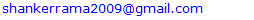Abstract

A Poisson-Akash distribution has been obtained by compounding Poisson distribution with Akash distribution introduced by Shanker (2015). A general expression for the th factorial moment has been derived and hence the first four moments about origin and the moments about mean has been obtained. The expressions for its coefficient of variation, skewness and kurtosis have been obtained. Its statistical properties including generating function, increasing hazard rate and unimodality and over-dispersion have been discussed. The maximum likelihood estimation and the method of moments for estimating its parameter have been discussed. The goodness of fit of the proposed distribution using maximum likelihood estimation has been given for some count data-sets and the fit is compared with that obtained by other distributions.

Keywords: Akash distribution, Compounding, Moments, Skewness, Kurtosis, Estimation of parameter, Statistical properties, Goodness of fit

Cite this paper: Rama Shanker, The Discrete Poisson-Akash Distribution, International Journal of Probability and Statistics , Vol. 6 No. 1, 2017, pp. 1-10. doi: 10.5923/j.ijps.20170601.01.

1. Introduction

A lifetime distribution named, “Akash distribution” having probability density function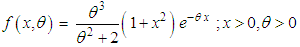(1.1)
has been proposed by Shanker (2015) for modeling lifetime data from biomedical science and engineering and shown that the proposed distribution is a two-component mixture of exponential distribution having scale parameter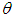and a gamma distribution having shape parameter 3 and scale parameterwith their mixing proportions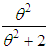and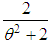respectively. Various mathematical and statistical properties of Akash distribution including its shape, moments, skewness, kurtosis, hazard rate function, mean residual life function, stochastic ordering, mean deviations, distribution of order statistics, Bonferroni and Lorenz curves, Renyi entropy measure and stress-strength reliability have been discussed by Shanker (2015). It has been shown by Shanker (2015) that Akash distribution provides much closer fit than Lindley and exponential distributions for modeling lifetime data from medical science and engineering. Further, Shanker et al (2016) have done a detailed comparative study on Akash, Lindley and exponential distributions for modeling different types of lifetime data from engineering and medical science and concluded that Akash distribution has some advantage over Lindley and exponential distributions, Lindley distribution has some advantage over Akash and exponential distributions and exponential distribution has some advantage over Akash and Lindley distributions due to their over-dispersion, equi-dispersion, and under-dispersion for various values of their parameters. Recently, Shanker and Shukla (2016) has introduced a two-parameter weighted Akash distribution (WAD) andstudied its various mathematical and statistical properties, estimation of parameter and application for modeling lifetime data. Shanker (2016) has also proposed a two-parameter quasi Akash distribution and studied its statistical and mathematical properties, estimation of parameters using maximum likelihood estimation and method of moments along with its application for modeling lifetime data from engineering and biomedical sciences.
In the present paper, a Poisson mixture of Akash distribution introduced by Shanker (2015) named, “Poisson-Akash distribution (PAD) has been proposed. Its various mathematical and statistical properties including its shape, moments, coefficient of variation, skewness, and kurtosis have been discussed. The estimation of its parameter has been discussed using maximum likelihood estimation and method of moments. The goodness of fit of PAD along with Poisson distribution and Poisson-Lindley distribution (PLD), a Poisson mixture of Lindley (1958) distribution and introduced by Sankaran (1970), has been given with some count data-sets.

2. Poisson-Akash Distribution

Suppose the parameter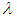of Poisson distribution follows Akash distribution (1.1). Then the Poisson mixture of Akash distribution (1.1) can be obtained as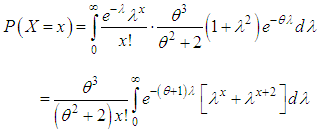(2.1)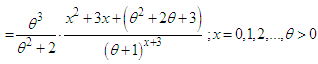(2.2)
We name this distribution “Poisson-Akash distribution (PAD)”. Shanker et al (2016) have detailed study on applications of PAD to model count data from different fields of knowledge.
It would be recalled that Sankaran (1970) obtained Poisson-Lindley distribution (PLD) having probability mass function (p.m.f)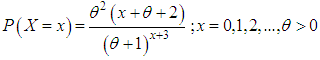(2.3)
by compounding Poisson distribution with Lindley distribution, introduced by Lindley (1958) having probability density function (p.d.f)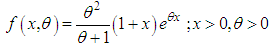(2.4)
Ghitany et al (2008) have discussed various interesting properties of Lindley distribution, estimation of parameter and application for modeling waiting time data from a bank. Shanker et al (2015) have detailed study on modeling of lifetime data using exponential and Lindley distributions. Further, Shanker et al (2016) have discussed the comparative applications of Akash, Lindley and exponential distributions for modeling lifetime data from biomedical sciences and engineering. Shanker and Hagos (2015) have detailed and critical study on applications of PLD to model count data from biological sciences.
The graphs of the pmf of Poisson-Akash distribution (PAD) and Poisson-Lindley distribution (PLD) for different values of their parameter are shown in the figure 1.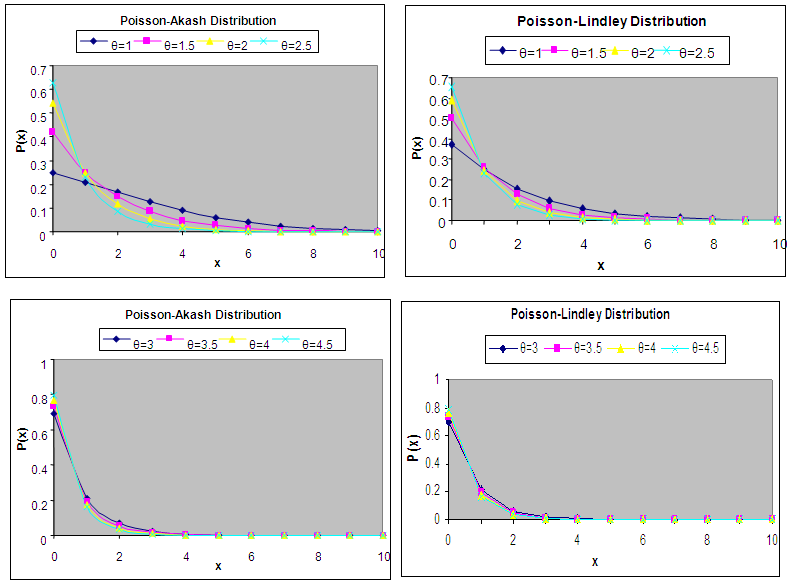Figure 1. Graphs of probability mass function of PAD and PLD for different values of the parameter θ

3. Moments and Associated Measures

The rth factorial moment about origin of Poisson-Akash distribution (PAD) (2.2) can be obtained as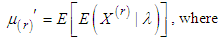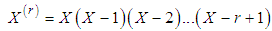Using (2.1), the rth factorial moment about origin of PAD (2.2) can be obtained as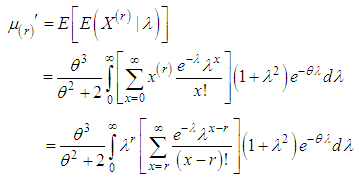Taking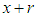in place of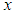within the bracket, we get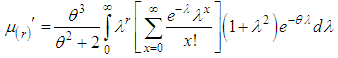The expression within the bracket is clearly unity and hence we have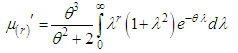Using gamma integral and a little algebraic simplification, we get finally, a general expression for the th factorial moment of PAD (2.2) as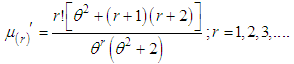(3.1)
Substituting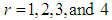in (3.1), the first four factorial moments about origin can be obtained and using the relationship between factorial moments about origin and moments about origin, the first four moment about origin of the PAD (2.2) are obtained as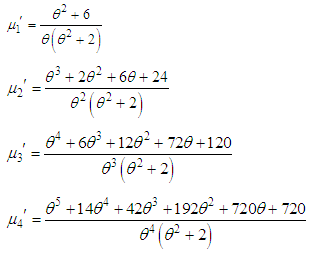Using the relationship between moments about mean and the moments about origin, the moments about mean of the PAD (2.2) are obtained as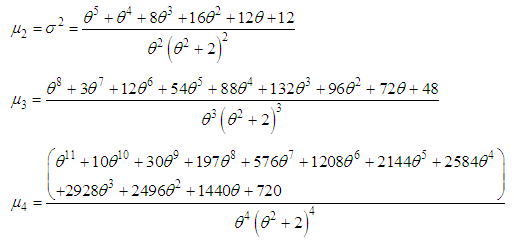The coefficient of variation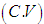, coefficient of skewness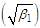, coefficient of kurtosis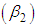, and index of dispersion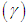of the PAD (2.2) are thus given by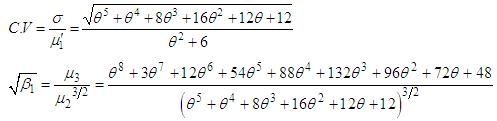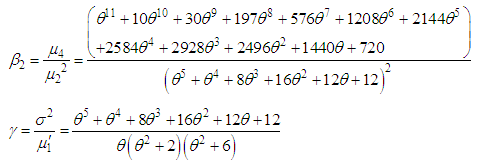The expressions for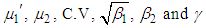of PLD (2.3) obtained by Sankaran (1970) and Ghitany and Al-Mutairi (2009) are given by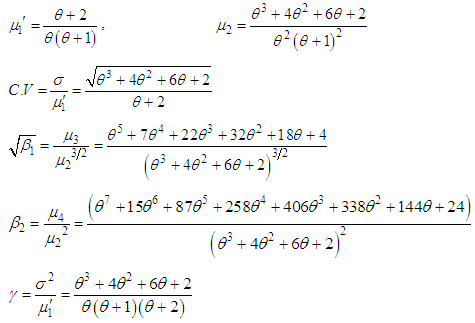Table 1. Values of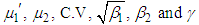of PAD and PLD for different values of θ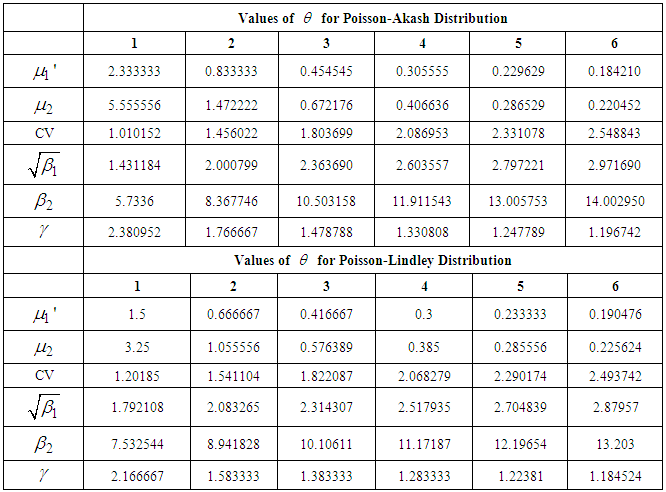To study the behavior of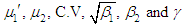of PAD and PLD, values of these characteristics for different values of parameter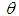have been computed and presented in table 1.
The graphs of coefficient of variation, coefficient of skewness, coefficient of kurtosis and index of dispersion of PAD and PLD for different values of the parameterhave been shown in the figure 2.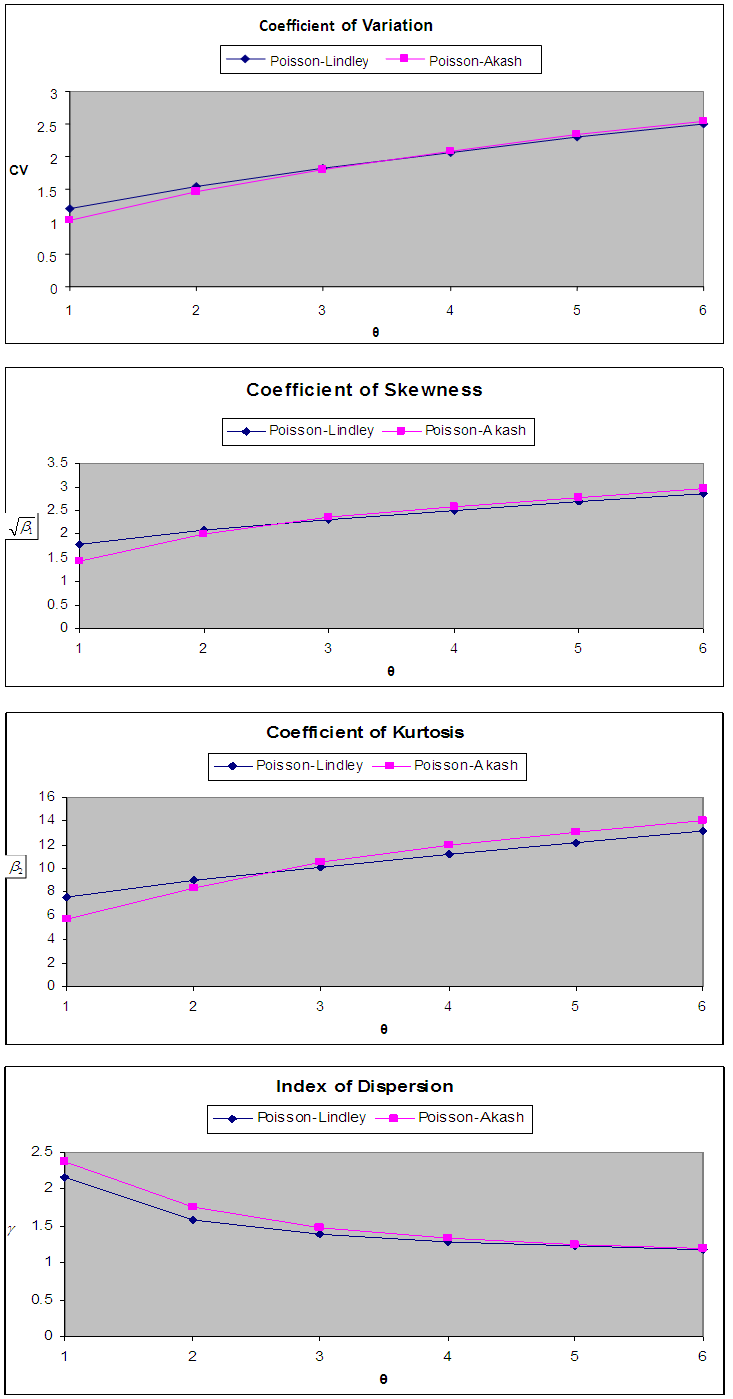Figure 2. Graphs of coefficient of variation, coefficient of skewness, coefficient of kurtosis and Index dispersion of PAD and PLD for different values of the parameter θ

4. Mathematical and Statistical Properties

4.1. Increasing Hazard Rate and Unimodality

The PAD (2.2) has an increasing hazard rate (IHR) and unimodal. Since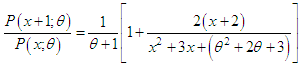is decreasing function in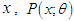is log-concave. Therefore, the PAD has an increasing hazard rate and unimodal. A detailed discussion about interrelationship between log-concavity, unimodality and increasing hazard rate (IHR) of discrete distributions can be seen in Grandell (1997).

4.2. Generating Functions

The probability generating function of PAD (2.2) can be obtained as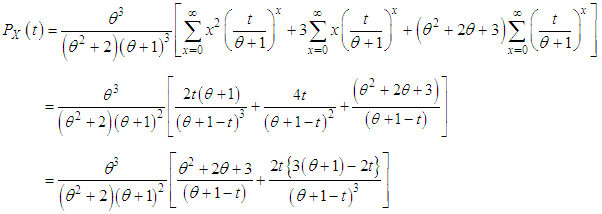The moment generating function of the PAD (2.2) can thus be obtained as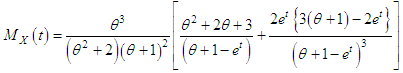4.3. Over-dispersion

The PAD (2.2) is always over-dispersed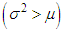. We have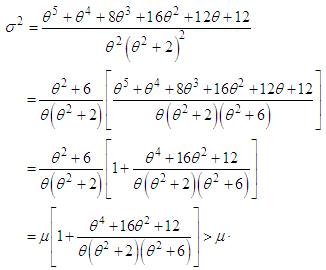This shows that PAD (2.2) is always over dispersed.

5. Estimation of the Parameter

5.1. Maximum Likelihood Estimate (MLE)

Let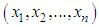be a random sample of size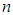from the PAD (2.2) and let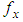be the observed frequency in the sample corresponding to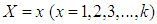such that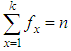, where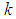is the largest observed value having non-zero frequency. The likelihood function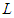of the PAD (2.2) is given by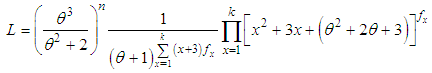The log likelihood function is thus obtained as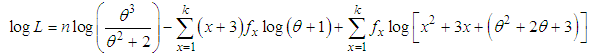The first derivative of the log likelihood function is given by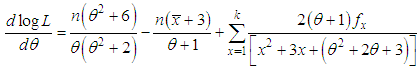where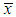is the sample mean.
The maximum likelihood estimate (MLE),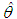of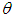of PAD (2.2) is the solution of the equation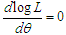and is given by the solution of the following non-linear equation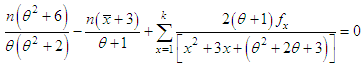This non-linear equation can be solved by any numerical iteration methods such as Newton- Raphson, Bisection method, Regula –Falsi method etc

5.2. Method of Moment Estimate (MOME)

Let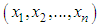be a random sample of size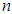from the PAD (2.2). Equating the population mean to the corresponding sample mean, the MOME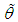ofof PAD (2.2) is the solution of the following cubic equation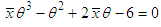whereis the sample mean.

6. Goodness of Fit

The PAD has been fitted to a number of data - sets to test its goodness of fit with Poisson distribution (PD) and Poisson-Lindley distribution (PLD. The maximum likelihood estimate (MLE) has been used to fit the PAD. Five examples of observed count data-sets, for which the PAD, PD, and PLD has been fitted, are presented. The first data-set is due to Kemp and kemp (1965) regarding the distribution of mistakes in copying groups of random digits, the second data-set is due to Beall (1940) regarding the distribution of Pyrausta nublilalis, the third data-set is the number of accidents to 647 women working on high explosive shells in 5 weeks, available in Sankaran (1970), the fourth data-set is the distribution of red mites per leaf on apple leaves, available in Fisher et al (1943), and the fifth data-set is distribution of number of Chromatid aberrations, available in Loeschke and Kohler (1976) and Janardan and Schaeffer (1977).
 Table 6.1. Distribution of mistakes in copying groups of random digits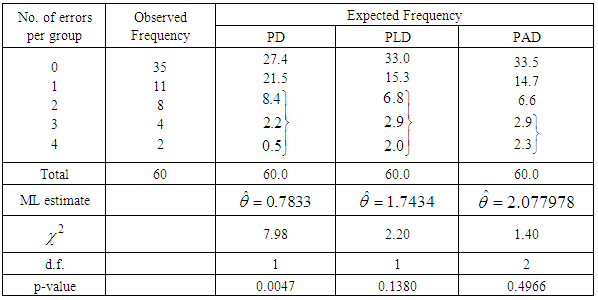Table 6.2. Distribution of Pyrausta nublilalis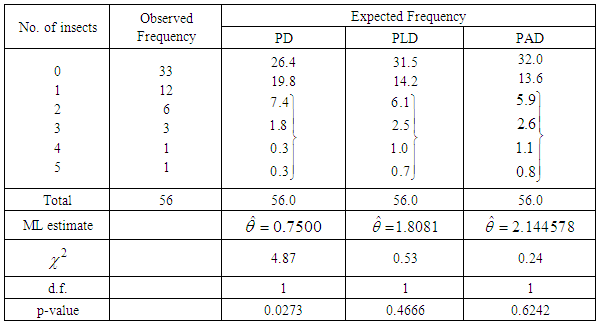Table 6.3. Accidents to 647 women working on high explosive shells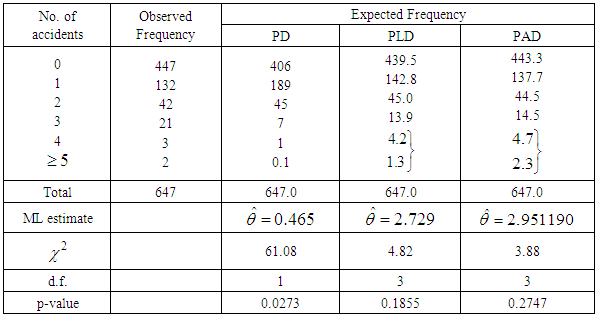Table 6.4. Distribution of number of red mites on Apple leaves, Fisher et al (1943)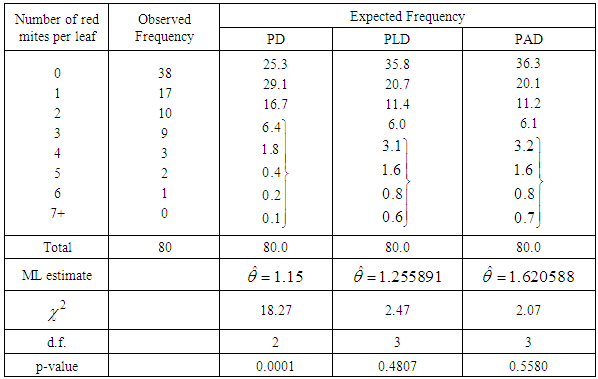Table 6.5. Distribution of number of Chromatid aberrations (0.2 g chinon 1, 24 hours)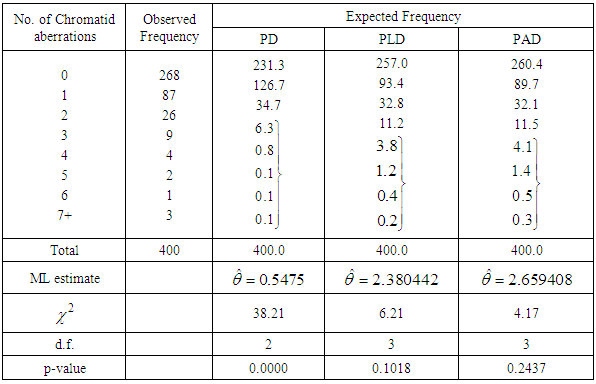7. Concluding Remarks

In this paper Poisson-Akash distribution (PAD) has been obtained by compounding Poisson distribution with Akash distribution introduced by Shanker (2015). The expression for the rth factorial moment has been derived and hence the first four moments about origin and the moments about mean has been given. The expression for coefficient of variation, skewness and kurtosis has been obtained. The maximum likelihood estimation and the method of moments for estimating its parameter have been discussed. The distribution has been fitted using maximum likelihood estimate to some data - sets to test its goodness of fit over Poisson distribution (PD) and Poisson-Lindley distribution (PLD) and it is clear from the fit of PAD that PAD gives much closer fit than PD and PLD in almost all data-sets.

ACKNOWLEDGEMENTS

The author is grateful to the editor-in-chief of the journal and the reviewer for constructive comments which lead to the improvement in the quality of the paper.

References

  Beall, G. (1940): The fit and significance of contagious distributions when applied to observations on larval insects, Ecology, 21, 460-474.  Fisher, R.A., Corpet, A.S. and Williams, C.B. (1943): The relation between the number of species and the number of individuals in a random sample of an animal population, Journal of Animal ecology, 12, 42 – 58.  Ghitany, M.E., Atieh, B. and Nadarajah, S (2008): Lindley distribution and its Application, Mathematics Computing and Simulation, 78, 493 – 506.  Ghitany, M.E. and Al-Mutairi, D.K. (2009): Estimation method for the discrete Poisson-Lindley distribution, Journal of Statistical Computation and Simulation, 79 (1), 1 – 9.  Grandell, J. (1997): Mixed Poisson Processes, Chapman& Hall, London.  Janardan, K.G. and Schaeffer, D.J. (1977): Models for the analysis of chromosomal aberrations in human leukocytes, Biometrical Journal, 19(8), 599 – 612.  Kemp, C.D. and Kemp, A.W. (1965): Some properties of the Hermite distribution, Biometrika, 52, 381-394.  Lindley, D.V. (1958): Fiducial distributions and Bayes theorem, Journal of the Royal Statistical Society, 20 (1), 102- 107.  Loeschke, V. and Kohler, W. (1976): Deterministic and Stochastic models of the negative binomial distribution and the analysis of chromosomal aberrations in human leukocytes, Biometrische Zeitschrift, 18, 427 -451.  Sankaran, M. (1970): The discrete Poisson-Lindley distribution, Biometrics, 26, 145- 149.  Shanker, R. (2015): Akash distribution and Its Applications, International Journal of Probability and Statistics, 4(3), 65 -75.  Shanker, R., Hagos, F. and Sujatha, S. (2016): On modeling of lifetimes data using one parameter Akash, Lindley and exponential distributions, Biometrics & Biostatistics International Journal, 3(2), 1 – 10.  Shanker, R. Hagos, F. and Teklay, T. (2016): On Poisson-Akash distribution and Its Applications, Biometrics & Biostatistics International Journal, 3(6), 1 – 9.  Shanker, R., Hagos, F. and Sujatha, S. (2015): On Modeling of Lifetimes data using Exponential and Lindley distributions: Biometrics & Biostatistics International Journal, 2(5), 1-9.  Shanker, R. and Hagos, F. (2015): On Poisson-Lindley distribution and its Applications to Biological Sciences, Biometrics & Biostatistics International Journal, 2(4), 1-5.  Shanker, R. and Shukla, K.K. (2016): Weighted Akash distribution and Its Application to model lifetime data, International Journal of Statistics, 39 (2), 1138 – 1147.  Shanker, R. (2016): A Quasi Akash Distribution To appear in Assam Statistical Review.